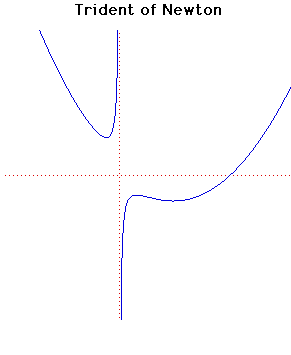# Curves

### Trident of NewtonCartesian equation:
$xy = cx^{3} + dx^{2} + ex + f$

### Description

This curve was investigated by Newton and also by Descartes. It is sometimes called the 'Parabola of Descartes' even although it is not a parabola. The name trident is due to Newton.

The curve occurs in Newton's study of cubics. It is contained in his classification of cubic curves which appears in Curves by Sir Isaac Newtonin Lexicon Technicumby John Harris published in London in 1710.

Newton was the first to undertake such a systematic study of cubic equations and he classified them into 72 different cases. In fact he missed six cases in his classification. The trident is the 66th species in his classification and Newton gives the graph essentially looking identical to the graph given above.

Newton states some properties of his trident. For example he states that the curve has four infinite legsand that the $y$-axis is an asymptote to two tending towards contrary parts .He also comments And this figure is that parabola by which D. Cartes (Descartes) constructed equations of six dimensions.

Newton's classification of cubics was criticised by Euler because it lacked general general principle. Plücker later gave a more detailed classification with 219 types.

### Associated Curves

Definitions of the Associated curves